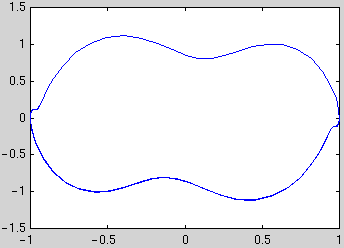# Using Matlab for Higher Order ODEs and Systems of ODEs

(Continuation of Using Matlab for First Order ODEs)

## Contents

Numerical Solution
Converting problems to first order systems
Plotting the solution
Finding numerical values at given t values
Making phase plane plots
Vector fields for autonomous problems
Plotting the vector field
Plotting the vector field together with solution curves
Symbolic Solution
Example with 2nd order problem
Plotting the solution
Finding numerical values at given t values
Example with first order system
Plotting the solution
Finding numerical values at given t values
Making phase plane plots

## Numerical solution

Example problem: The angle y of an undamped pendulum with a driving force sin(5 t) satisfies the differential equation

y'' = -sin(y) + sin(5 t)

and the initial conditions

y(0) = 1
y'(0) = 0.

If your problem is of order 2 or higher: rewrite your problem as a first order system. Let y1=y and y2=y', this gives the first order system

y1' = y2,
y2' = -sin(y1) + sin(5 t)

with the initial conditions

y1(0) = 1
y2(0) = 0.

Define an @-function `f` for the right hand side of the first order system: Note that `f` must be specified as a column vector using `[`...`;`...`]` (not a row vector using `[`...`,`...]). In the definition of `f`, use `y(1)` for y1, use `y(2)` for y2. The definition of `f` should have the form

`f = @(t,y) [`expression for y1' `;` expression for y2' `];`

You have to use `f = @(t,y)...` even if `t` does not occur in your formula. For our example the first component of `f` is y2, the second component is -sin(y1) + sin(5 t) and we define

`f = @(t,y) [y(2); -sin(y(1))+sin(5*t)] `

To plot the numerical solution: To obtain plots of all the components of the solution for t going from t0 to t1 use `ode45(f,[t0,t1],[y10;y20])` where `y10`, `y20` are the initial values for y1, y2 at the starting point `t0`. In our example we get a plot for t going from 0 to 20 with the initial values `[1;0]` using

`ode45(f,[0,20],[1;0])`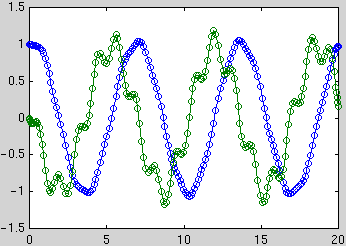This shows the two functions y1(t)=y(t) (blue) and y2(t)=y'(t) (green).

The circles mark the values which were actually computed (the points are chosen by Matlab to optimize accuracy and efficiency). You can obtain a vector `ts` and a matrix `ys` with the coordinates of these points using ```[ts,ys] = ode45(f,[t0,t1],[y10;y20])```. You can then plot the solution curves using `plot(ts,ys)` (this is a way to obtain a plot without the circles). Note that each row of the matrix `ys` contains 2 entries corresponding to the two components of the solution at that time:

```[ts,ys] = ode45(f,[0,20],[1;0]); % find ts, ys, but don't show plot(ts,ys) % make plot of y1 and y2 vs. t [ts,ys] % show table with 3 columns for t, y1, y2```

You can obtain the vector of `y1` values in the first column of `ys` by using `ys(:,1)`, therefore `plot(ts,ys(:,1))` plots only y1(t).

To obtain numerical values at specific t values: You can specify a vector `tv` of t values and use ```[ts,ys] = ode45(f,tv,[y10;y20])```. The first element of the vector `tv` is the initial t value; the vector `tv` must have at least 3 elements. E.g., to obtain the solution with the initial values `[1;0]` at t = 0, 0.5, ..., 10 and display the results as a table with 3 columns t, y1, y2, use

```[ts,ys]=ode45(f,0:0.5:10,[1;0]); [ts,ys]```

To plot trajectories in the phase plane: To see the points with coordinates ( y1(t), y2(t) ) in the y1, y2 plane for t going from 0 to 20 type

```options=odeset('OutputFcn','odephas2'); ode45(f,[0,20],[1;0],options)```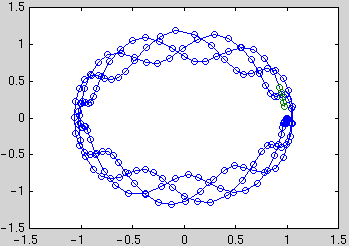This shows the points while they are being computed (the plotting can be stopped with the stop button). To first compute the numerical values and then plot the curve without the circles, type

```[ts,ys] = ode45(f,[0,20],[1;0]); % find ts, ys, but don't show plot(ys(:,1),ys(:,2)) % make plot of y2 vs. y1```

## Vector fields for autonomous systems of two first order ODEs

If the right hand side function f(t, y) does not depend on t, the problem is called autonomous. In this case the behavior of the differential equation can be visualized by plotting the vector f(t, y) at each point y = (y1,y2) in the y1,y2 plane (the so-called phase plane).

First save the files `vectfield.m` and `vectfieldn.m` into the same directory where your m-files are.

To plot the vector field for y1 going from a1 to b1 with a spacing of d1 and y2 going from a2 to b2 with a spacing of d2 use `vectfield(f,a1:d1:b1,a2:d2:b2)`. The command `vectfieldn` works in the same way, but produces arrows which all have the same length. This makes it easier to see the direction of the vector field.

Example: The undamped pendulum problem without a driving force has the differential equation y'' = -sin(y). This gives the first order system

y1' = y2,
y2' = -sin(y1)

Here we define

`f = @(t,y) [y(2);-sin(y(1))] `

We can plot the vector field and 10 trajectories with starting points (0,0), (0,0.3), ..., (0,2.7) in the phase plane as follows:

```vectfield(f,-2:.5:8,-2.5:.25:2.5)
hold on
for y20=0:0.3:2.7
[ts,ys] = ode45(f,[0,10],[0;y20]);
plot(ys(:,1),ys(:,2))
end
hold off```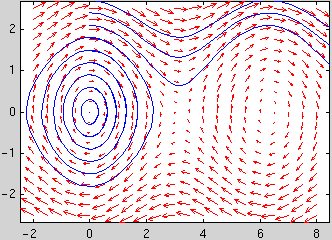## Symbolic solution

Use the `dsolve` command. Specify all differential equations as strings, using `Dy` for y'(t), `D2y` for y''(t) etc. .

For an initial value problem specify the initial conditions in the form `'y(t0)=y0'`, `'Dy(t0)=y1'` etc. . The last argument of `dsolve` is the name of the independent variable, e.g., `'t'`.

Example: For the differential equation

y'' = -y + sin(5 t)

use

`sol = dsolve('D2y = -y + sin(5*t)','t')`

In this case, the answer can be simplified by typing

`s = simple(sol)`

which gives the general solution `-1/24*sin(5*t)+C1*cos(t)+C2*sin(t)` with two constants `C1`, `C2`.

To solve the ODE with initial conditions y(0) = 1, y'(0) = 0 use

```sol = dsolve('D2y = -y + sin(5*t)','y(0)=1','Dy(0)=0','t')```

Then `s = simple(sol)` gives the solution `-1/24*sin(5*t)+5/24*sin(t)+cos(t)`.

To plot the solution curve use `ezplot`:

```ezplot(sol, [0,20])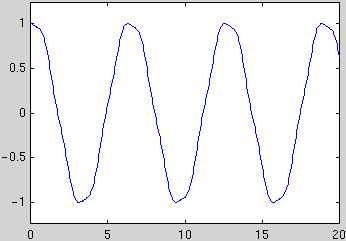```

Example for system of ODEs: For the system

y1' = y2,
y2' = -y1 + sin(5 t)

with the initial conditions

y1(0) = 1
y2(0) = 0

type

```sol = dsolve('Dy1=y2','Dy2=-y1+sin(5*t)','y1(0)=1','y2(0)=0','t')```

which gives the somewhat strange response

```sol = y1: [1x1 sym] y2: [1x1 sym]```

This means that the two components of the solution can be accessed as `sol.y1` and `sol.y2` : Typing

`sol.y1`

gives `-2/3*sin(t)*cos(t)^4+1/2*sin(t)*cos(t)^2+1/6*sin(t)+cos(t)`. This can be simplified by typing

`s1 = simple(sol.y1)`

which gives `-1/24*sin(5*t)+5/24*sin(t)+cos(t)`. For the second component `y2` of the solution we proceed in the same way: Typing

`s2 = simple(sol.y2)`

gives `-sin(t)-5/24*cos(5*t)+5/24*cos(t)`.

To plot the solution curves use `ezplot`:

```ezplot(sol.y1, [0,20]) hold on ezplot(sol.y2, [0,20]) hold off```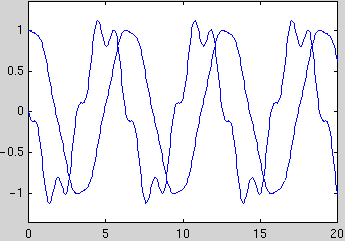To obtain numerical values use `double(subs(sol.y1,'t',tval))` where `tval` is a number or a vector of numbers. E.g., the following commands generate a table with three columns `t`, `y1`, `y2` for t=0, 0.5, ..., 10:

```tval = (0:0.5:10)'; % column vector with t-values yval = double(subs([sol.y1,sol.y2],'t',tval)); % 2 columns with y1,y2 [tval, yval] % display 3 columns together```

To plot the solution in the phase plane use a similar approach with more t values:

```tval = (0:0.1:10)'; % column vector with t-values yval = double(subs([sol.y1,sol.y2],'t',tval)); % 2 columns with y1,y2 plot(yval(:,1),yval(:,2)) % plot col.2 of yval vs. col.1 of yval```